# Unleashing the Power of Date-Related Functions in Salesforce Formulas: Streamlining Business Logic

Introduction:

Salesforce formulas offer a multitude of functions to handle date-related calculations, making them an essential tool for implementing business logic. From calculating durations to determining deadlines, leveraging date-related functions can significantly enhance automation and decision-making processes. In this blog post, we will explore four complex scenarios where date-related functions prove invaluable and provide step-by-step explanations on how to implement them effectively.

Understanding Important Date-Related Functions in Formulas:

Salesforce provides a comprehensive set of date-related functions to handle various calculations and manipulations. Here are some of the key functions you need to be familiar with:

• TODAY(): Returns the current date in the user's time zone.
• DATE(year, month, day): Constructs a date using the provided year, month, and day values.
• DATEVALUE(dateString): Converts a date string into a Salesforce date value.
• DATETIMEVALUE(dateTimeString): Converts a date-time string into a Salesforce date-time value.
• YEAR(date): Returns the year component of a date or date-time value.
• MONTH(date): Returns the month component of a date or date-time value.
• DAY(date): Returns the day component of a date or date-time value.
• ADDYEARS(date, numYears): Adds the specified number of years to a date value.
• ADDMONTHS(date, numMonths): Adds the specified number of months to a date value.
• ADDWEEKS(date, numWeeks): Adds the specified number of weeks to a date value.
• ADDDAYS(date, numDays): Adds the specified number of days to a date value.

Now, let's delve into the complex scenarios where date-related functions can be utilized effectively in Salesforce formulas:

Scenario 1: Calculating Project Duration

In project management, determining the duration between two dates is crucial. Let's say you have a custom object called "Project" with "Start_Date__c" and "End_Date__c" fields. You can calculate the duration between these dates using the `DAY()` function and perform additional calculations if necessary.

Implementation: End_Date__c - Start_Date__c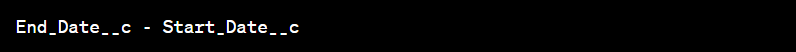In task management, flagging overdue tasks is essential for prompt action. Assume you have a custom object called "Task" with a "Due_Date__c" field. You can use the `TODAY()` function to compare the due date with the current date and set a flag if the task is overdue.

Implementation:
IF(TODAY() > Due_Date__c, "Overdue", "On Track")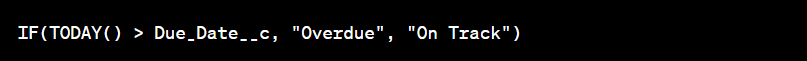Scenario 3: Calculating Time Remaining for SLA Compliance

Service Level Agreements (SLAs) often require tracking the time remaining for compliance. Suppose you have a custom object called "Case" with a "SLA_Due_Date__c" field. You can calculate the time remaining until the SLA deadline using the `TODAY()` and `DAY()` functions.

Implementation:
SLA_Due_Date__c - TODAY()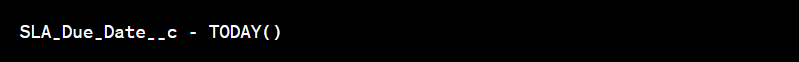Scenario 4: Estimating Project Completion Date

Predicting project completion dates based on the current progress and expected effort is crucial for effective project management. Let's consider a custom object called "Project" with fields like "Start_Date__c," "Estimated_Duration__c," and "Actual_Duration__c." You can calculate the estimated project completion date using the `ADDWEEKS()` or `ADDDAYS()` functions.

Implementation: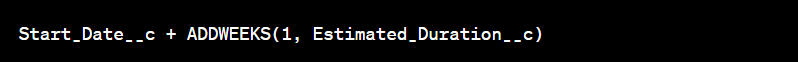Scenario 5: Tracking Employee Tenure and Awarding Vacation Days

In human resources management, tracking employee tenure and awarding vacation days based on the length of service is a common requirement. You can leverage date-related formulas to calculate the tenure of employees in years and determine the number of vacation days they are eligible for.

Implementation:
Assume you have a custom object called "Employee" with a "Hire_Date__c" field to capture the date of employee hire. Here's how you can implement the calculation for tenure and vacation days:

1. Calculating Tenure in Years:
To calculate the tenure in years, you can use the `YEAR()` and `MONTH()` functions to determine the difference between the current date and the hire date, considering both the year and month components.

YEAR(TODAY()) - YEAR(Hire_Date__c) - IF(MONTH(TODAY()) < MONTH(Hire_Date__c), 1, 0)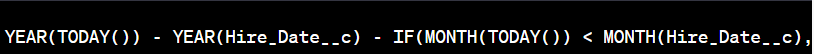This formula subtracts the hire year from the current year and adjusts the result by subtracting 1 if the current month is earlier than the hire month. This accurately calculates the tenure in years, accounting for both year and month differences.

2. Awarding Vacation Days:

Once you have calculated the tenure in years, you can use an IF statement to determine the number of vacation days an employee is eligible for based on your organization's vacation policy.

IF(Tenure__c < 5, 15, IF(Tenure__c < 10, 20, 25))This formula checks the employee's tenure (`Tenure__c` field) and assigns the appropriate number of vacation days. In this example, employees with less than 5 years of tenure receive 15 vacation days, those with 5 to 9 years receive 20 days, and employees with 10 or more years receive 25 days.

By combining these formulas, you can track employee tenure accurately and dynamically award vacation days based on their length of service.

Conclusion:

Date-related functions in Salesforce formulas are powerful tools for implementing business logic. In this blog post, we explored four complex scenarios where these functions can be utilized effectively, ranging from calculating project durations to estimating completion dates. By leveraging functions such as `TODAY()`, `ADDYEARS()`, and `DAY()`, you can automate date calculations, streamline decision-making processes, and enhance productivity in your Salesforce org.

In the final scenario discussed above, we demonstrated how to calculate tenure in years using the `YEAR()` and `MONTH()` functions, and how to determine the number of vacation days using an IF statement based on tenure. These formulas enable organizations to automate tenure tracking, streamline vacation policy enforcement, and ensure accurate entitlements for employees.

Remember to consult Salesforce documentation for a comprehensive list of date-related functions and best practices to leverage their full potential. Empower your organization with the capabilities of date-related functions and unlock new levels of efficiency and accuracy in your Salesforce implementation.